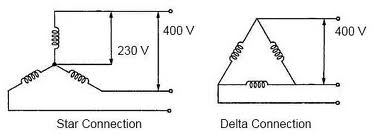## Voltage - Current and Power Relations Assignment Help

Assignment Help: >> General Notations used in 3-phase - Voltage - Current and Power Relations

Voltage, Current and Power Relations:

In Star Connection

Vph = Phase voltage VL = Line voltage Iph  = Phase current IL = Line currentFigure

In star connection

VL = √3 Vph

I L = I ph

P = √3 VL I L cos θ Watts

= 3 V ph I ph cos θ

Where cos θ = Power factor.

In Delta Connection

VL = V ph

I L  = √3 I ph

P = 3 VL I L cos θ Watts

= 3 V ph I ph cos θ